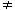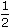# Aptitude - Logarithm

Exercise : Logarithm - General Questions
1.
Which of the following statements is not correct?
log10 10 = 1
log (2 + 3) = log (2 x 3)
log10 1 = 0
log (1 + 2 + 3) = log 1 + log 2 + log 3
Explanation:

(a) Since loga a = 1, so log10 10 = 1.

(b) log (2 + 3) = log 5 and log (2 x 3) = log 6 = log 2 + log 3log (2 + 3)log (2 x 3)

(c) Since loga 1 = 0, so log10 1 = 0.

(d) log (1 + 2 + 3) = log 6 = log (1 x 2 x 3) = log 1 + log 2 + log 3.

So, (b) is incorrect.

2.
If log 2 = 0.3010 and log 3 = 0.4771, the value of log5 512 is:
2.870
2.967
3.876
3.912
Explanation:
log5 512
 = log 512 log 5
 = log 29 log (10/2)
 = 9 log 2 log 10 - log 2
 = (9 x 0.3010) 1 - 0.3010
 = 2.709 0.699
 = 2709 699
= 3.876

3.
 log 8 is equal to: log 8
 1 8
 1 4
 1 2
 1 8
Explanation:
 log 8 = log (8)1/2 =log 8 = 1 . log 8 log 8 log 8 2

4.
If log 27 = 1.431, then the value of log 9 is:
0.934
0.945
0.954
0.958
Explanation:

log 27 = 1.431log (33 ) = 1.4313 log 3 = 1.431log 3 = 0.477log 9 = log(32 ) = 2 log 3 = (2 x 0.477) = 0.954.

5.
 If log a + log b = log (a + b), then: b a
a + b = 1
a - b = 1
a = b
a2 - b2 = 1log (a + b) = loga x b= log 1. b a